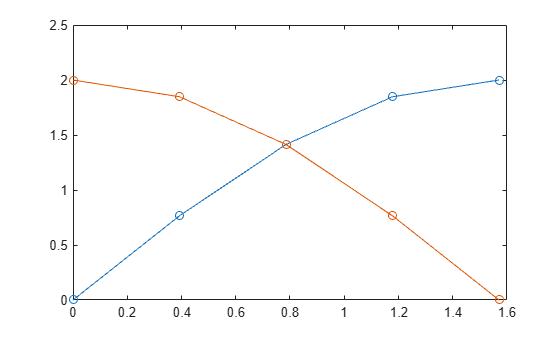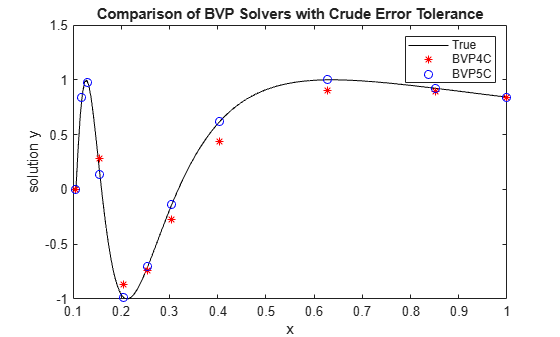# bvp5c

## 语法

``sol = bvp5c(odefun,bcfun,solinit)``
``sol = bvp5c(odefun,bcfun,solinit,options)``

## 说明

``sol = bvp5c(odefun,bcfun,solinit)` 使用 `bcfun` 描述的边界条件和初始解估计值 `solinit` 对 `odefun` 指定的形式为 y′ = f(x,y) 的微分方程组进行积分。使用 `bvpinit` 函数创建初始估计值 `solinit`，该函数还定义了要强制应用 `bcfun` 中边界条件的点。`

``sol = bvp5c(odefun,bcfun,solinit,options)` 还使用由 `options`（使用 `bvpset` 函数创建的参数）定义的积分设置。例如，使用 `AbsTol` 和 `RelTol` 选项指定绝对误差容限和相对误差容限，或者使用 `FJacobian` 选项提供 `odefun` 的解析偏导数。`

## 示例

${{\mathit{y}}^{\prime }}^{\prime }+\mathit{y}=0$.

$\mathit{y}\left(0\right)=0$,

$\mathit{y}\left(\pi /2\right)=2$.

${{\mathit{y}}_{1}}^{\prime }={\mathit{y}}_{2}$,

${{\mathit{y}}_{2}}^{\prime }=-{\mathit{y}}_{1}$.

```function dydx = bvpfcn(x,y) dydx = zeros(2,1); dydx = [y(2) -y(1)]; end ```

$\mathit{y}\left(0\right)=0$,

$\mathit{y}\left(\pi /2\right)-2=0$.

```function res = bcfcn(ya,yb) res = [ya(1) yb(1)-2]; end ```

```function g = guess(x) g = [sin(x) cos(x)]; end ```
```xmesh = linspace(0,pi/2,5); solinit = bvpinit(xmesh, @guess);```

`sol = bvp5c(@bvpfcn, @bcfcn, solinit);`

`plot(sol.x, sol.y, '-o')````function dydx = bvpfcn(x,y) % equation to solve dydx = zeros(2,1); dydx = [y(2) -y(1)]; end %-------------------------------- function res = bcfcn(ya,yb) % boundary conditions res = [ya(1) yb(1)-2]; end %-------------------------------- function g = guess(x) % initial guess for y and y' g = [sin(x) cos(x)]; end %--------------------------------```

${{\mathit{y}}^{\prime }}^{\prime }+\frac{2}{\mathit{x}}{\mathit{y}}^{\prime }+\frac{1}{{\mathit{x}}^{4}}\mathit{y}=0$.

$\mathit{y}\left(\frac{1}{3\pi }\right)=0$,

$\mathit{y}\left(1\right)=\mathrm{sin}\left(1\right)$

${{\mathit{y}}_{1}}^{\prime }={\mathit{y}}_{2}$,

${{\mathit{y}}_{2}}^{\prime }=-\frac{2}{\mathit{x}}{\mathit{y}}_{2}-\frac{1}{{\mathit{x}}^{4}}{\mathit{y}}_{1}$

```function dydx = bvpfcn(x,y) dydx = [y(2) -2*y(2)/x - y(1)/x^4]; end ```

$\mathit{y}\left(\frac{1}{3\pi }\right)=0$,

$\mathit{y}\left(1\right)-\mathrm{sin}\left(1\right)=0$

```function res = bcfcn(ya,yb) res = [ya(1) yb(1)-sin(1)]; end ```

$\mathit{J}=\frac{\partial {\mathit{f}}_{\mathit{i}}}{\partial \mathit{y}}=\left[\begin{array}{cc}\frac{\partial {\mathit{f}}_{1}}{\partial {\mathit{y}}_{1}}& \frac{\partial {\mathit{f}}_{1}}{\partial {\mathit{y}}_{2}}\\ \frac{\partial {\mathit{f}}_{2}}{\partial {\mathit{y}}_{1}}& \frac{\partial {\mathit{f}}_{2}}{\partial {\mathit{y}}_{2}}\end{array}\right]=\left[\begin{array}{cc}\text{}\text{}\text{}\text{}0& \text{}\text{}\text{}\text{}1\\ -\frac{1}{{\mathit{x}}^{4}}& -\frac{2}{\mathit{x}}\end{array}\right]$

```function dfdy = jac(x,y) dfdy = [0 1 -1/x^4 -2/x]; end ```
`opts = bvpset('FJacobian',@jac,'RelTol',0.1,'AbsTol',0.1,'Stats','on');`

```xmesh = linspace(1/(3*pi), 1, 10); solinit = bvpinit(xmesh, [1; 1]);```

`bvp4c``bvp5c` 分别求解方程。

`sol4c = bvp4c(@bvpfcn, @bcfcn, solinit, opts);`
```The solution was obtained on a mesh of 9 points. The maximum residual is 9.794e-02. There were 157 calls to the ODE function. There were 28 calls to the BC function. ```
`sol5c = bvp5c(@bvpfcn, @bcfcn, solinit, opts);`
```The solution was obtained on a mesh of 11 points. The maximum error is 6.742e-02. There were 244 calls to the ODE function. There were 29 calls to the BC function. ```

${\mathit{y}}_{1}=\mathrm{sin}\left(\frac{1}{\mathit{x}}\right)$,

${\mathit{y}}_{2}=-\frac{1}{{\mathit{x}}^{2}}\mathrm{cos}\left(\frac{1}{\mathit{x}}\right)$.

```xplot = linspace(1/(3*pi),1,200); yplot = [sin(1./xplot); -cos(1./xplot)./xplot.^2]; plot(xplot,yplot(1,:),'k',sol4c.x,sol4c.y(1,:),'r*',sol5c.x,sol5c.y(1,:),'bo') title('Comparison of BVP Solvers with Crude Error Tolerance') legend('True','BVP4C','BVP5C') xlabel('x') ylabel('solution y')``````function dydx = bvpfcn(x,y) % equation to solve dydx = [y(2) -2*y(2)/x - y(1)/x^4]; end %--------------------------------- function res = bcfcn(ya,yb) % boundary conditions res = [ya(1) yb(1)-sin(1)]; end %--------------------------------- function dfdy = jac(x,y) % analytical jacobian for f dfdy = [0 1 -1/x^4 -2/x]; end %---------------------------------```

## 输入参数

```function dydt = odefun(t,y) dydt = 5*y-3; end```

`$\begin{array}{l}y{\text{'}}_{1}={y}_{1}+2{y}_{2}\\ y{\text{'}}_{2}=3{y}_{1}+2{y}_{2}\end{array}$`

```function dydt = odefun(t,y) dydt = zeros(2,1); dydt(1) = y(1)+2*y(2); dydt(2) = 3*y(1)+2*y(2); end```

`bvp5c` 也可以求解解具有奇异性或具有多点边界条件的问题。

#### 未知参数

```function res = bcfun(ya,yb) res = [ya(1)-1 yb(1)]; end```

#### 未知参数

options 结构体。使用 `bvpset` 函数创建或修改 options 结构体。

## 输出参数

`x`

`bvp5c` 选择的网格。此网格通常包含不同于初始网格 `solinit.x` 的点。

`y`

`sol.x` 网格点处的 y(x) 近似值。

`yp`

`sol.x` 网格点处的 y′(x) 近似值。

`parameters`

`solinit.parameters` 中指定的未知参数的最终值。

`solver`

`'bvp5c'`

`stats`

## 详细信息

### 多点边界值问题

`bvp5c` 可以求解多点边界值问题（其中 a = a0 < a1 < a2 < ...< an = b 是区间 [a,b] 中的边界点）。点 a1,a2,...,an−1 表示将 [a,b] 分为多个区域的界点。`bvp5c` 使用从 1 开始的索引按从左到右的顺序（从 a 到 b）枚举区域。在区域 k [ak−1,ak] 中，`bvp5c` 按如下所示计算导数

`yp = odefun(x,y,k) `

### 奇异边界值问题

`bvp5c` 对一类奇异边界值问题求解，其中包括具有未知参数 `p` 的形式如下的问题

`$\begin{array}{l}y\text{'}=S\frac{y}{x}+f\left(x,y,p\right),\\ 0=bc\left(y\left(0\right),y\left(b\right),p\right).\end{array}$`

## 算法

`bvp5c` 是一个有限差分代码，此代码实现 4 阶段 Lobatto IIIa 公式 。这是配置公式，并且配置多项式会提供在整个 `[a,b]` 中具有一致五阶精度的 CC1 连续解。该公式作为隐式 Runge-Kutta 公式实现。`bvp5c``bvp4c` 之间的一些差异是：

• `bvp5c` 直接求解代数方程。`bvp4c` 使用解析凝聚。

• `bvp4c` 直接处理未知参数。`bvp5c` 使用未知参数的平凡微分方程扩充方程组。

 Shampine, L.F., and J. Kierzenka. "A BVP Solver that Controls Residual and Error." J. Numer. Anal. Ind. Appl. Math. Vol. 3(1-2), 2008, pp. 27–41.